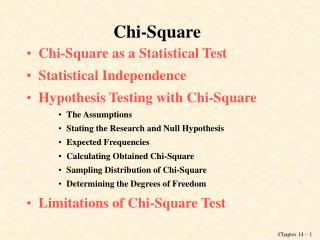DownloadDownload PresentationChi-Square

# Chi-Square

Download Presentation## Chi-Square

- - - - - - - - - - - - - - - - - - - - - - - - - - - E N D - - - - - - - - - - - - - - - - - - - - - - - - - - -
##### Presentation Transcript

1. Chi-Square • Chi-Square as a Statistical Test • Statistical Independence • Hypothesis Testing with Chi-Square • The Assumptions • Stating the Research and Null Hypothesis • Expected Frequencies • Calculating Obtained Chi-Square • Sampling Distribution of Chi-Square • Determining the Degrees of Freedom • Limitations of Chi-Square Test

2. Chi-Square as a Statistical Test • Chi-square test: an inferential statistics technique designed to test for significant relationships between two variables organized in a bivariate table. • Chi-square requires no assumptions about the shape of the population distribution from which a sample is drawn. • It can be applied to nominally or ordinallymeasured variables.

3. Gender and Fear of Walking Alone at Night Gender and Fear are associated but can this relationship be generalized to the population?

4. Statistical Independence • Independence (statistical): the absence of association between two cross-tabulated variables. The percentage distributions of the dependent variable within each category of the independent variable are identical.

5. Gender and Fear of Walking Alone at Night Gender and Fear are not related

6. Hypothesis Testing with Chi-Square Chi-square follows five steps: • Making assumptions • Stating the research and null hypotheses and selecting alpha • Selecting the sampling distribution and specifying the test statistic • Computing the test statistic • Making a decision and interpreting the results

7. The Assumptions • The chi-square test requires no assumptions about the shape of the population distribution from which the sample was drawn. • However, like all inferential techniques it assumes random sampling. • It can be applied to variables measured at a nominal and/or an ordinal level of measurement.

8. Stating Research and Null Hypotheses • The research hypothesis (H1) proposes that the two variables are related in the population. • The null hypothesis (H0) states that no association exists between the two cross-tabulated variables in the population, and therefore the variables are statistically independent.

9. H1:The two variables are related in the population. Gender and fear of walking alone at night are statistically dependent. H0:There is no association between the two variables. Gender and fear of walking alone at night are statistically independent.

10. The Concept of Expected Frequencies Expected frequencies fe :the cell frequencies that would be expected in a bivariate table if the two tables were statistically independent. Observed frequencies fo: the cell frequencies actually observed in a bivariate table.

11. Calculating Expected Frequencies fe = (column marginal)(row marginal) N To obtain the expected frequencies for any cell in any cross-tabulation in which the two variables are assumed independent, multiply the row and column totals for that cell and divide the product by the total number of cases in the table.

12. Gender and Fear -Observed and Expected Frequencies ((24)*(35))/59=14

13. Calculating the Obtained Chi-Square fe= expected frequencies fo = observed frequencies

14. Calculating the Obtained Chi-Square

15. The Sampling Distribution of Chi-Square • The sampling distribution of chi-square tells the probability of getting values of chi-square, assuming no relationship exists in the population. • The chi-square sampling distributions depend on the degrees of freedom. • The  sampling distribution is not one distribution, but is a family of distributions.

16. The Sampling Distribution of Chi-Square • The distributions are positively skewed. The research hypothesis for the chi-square is always a one-tailed test. • Chi-square values are always positive. The minimum possible value is zero, with no upper limit to its maximum value. • As the number of degrees of freedom increases, the  distribution becomes more symmetrical.

17. Determining the Degrees of Freedom df = (r – 1)(c – 1) where r = the number of rows c = the number of columns

18. Calculating Degrees of Freedom How many degrees of freedom would a table with 3 rows and 2 columns have? (3 – 1)(2 – 1) = 2 2 degrees of freedom

19. The Sampling Distribution of Chi-square-Appendix D Our obtained Chi Square > than 10.827 with p =.001. Thus our Chi-Square would occur even less than that if H0 was true. We reject H0 at the .001 level.

20. Limitations of the Chi-Square Test • The chi-square test does not give us much information about the strength of the relationship or its substantive significance in the population. • The chi-square test is sensitive to sample size. The size of the calculated chi-square is directly proportional to the size of the sample, independent of the strength of the relationship between the variables. • The chi-square test is also sensitive to small expected frequencies in one or more of the cells in the table.